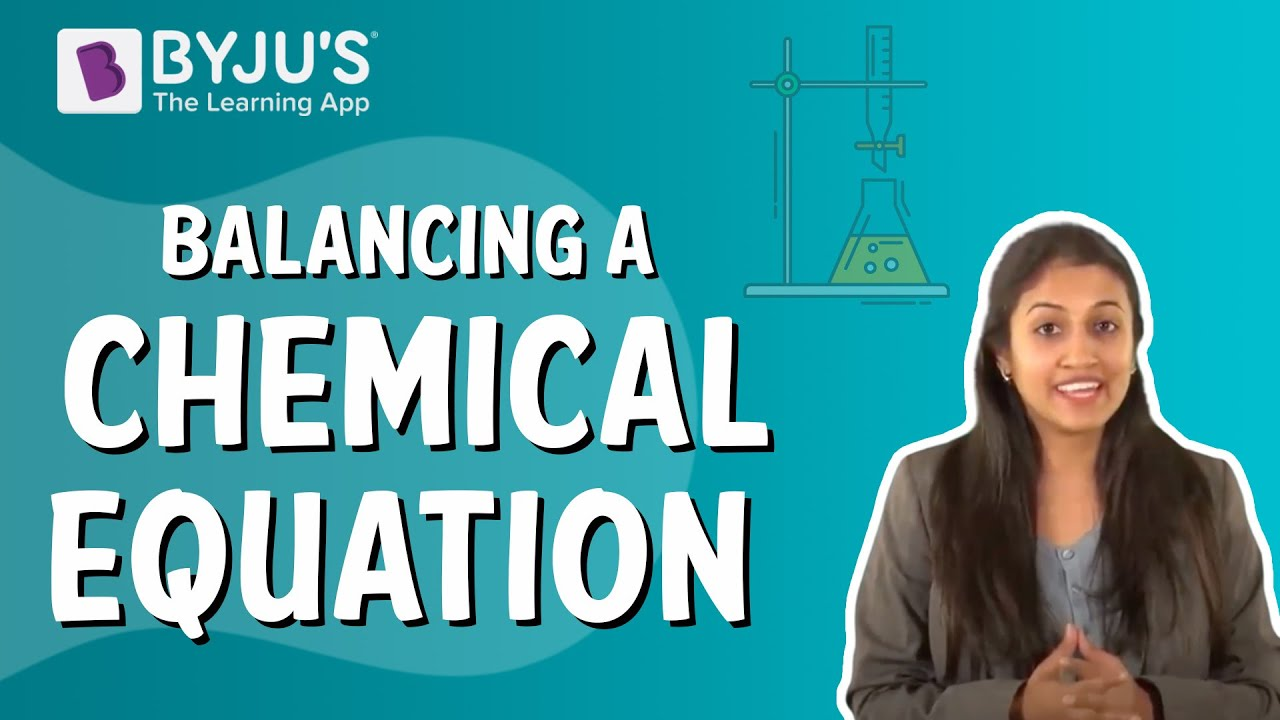# Balance the reaction. Fe2O3 + CO → Fe + CO2

The representation of a chemical reaction in the form of substances is known as a chemical equation. The equation in which the number of atoms of all the molecules is equal on both sides of the equation is known as a balanced chemical equation.

The given equation is

Fe2O3 + CO → Fe + CO2

To balance the given equation we have to note the number of atoms present om both sides

 Atom Reactant Side Product Side Fe 2 1 O 4 2 C 1 1

From the above table, we note that

• There are two iron atoms on the reactant side. So, we have to add coefficient 2 before iron on the product side
• There are four oxygen atoms on the reactant side. So, we have to add a coefficient of 2 before carbon dioxide on the product side.

Fe2O3 + CO → 2Fe + 2CO2

• To make the carbon atoms equal let us add coefficient 3 on both sides of the reaction.

The balanced chemical equation is

Fe2O3 + 3CO → 2Fe + 3CO2
Check out the video given below to know how to balance chemical equationsArticles to explore(302)(56)